# Electronics and Communication Engineering - Exam Questions Papers

26.

Find E at P(1, 1, 1) caused by four identical 3 nC point charges located at P1(1, 1, 0), P2(- 1, 1, 0), P3(- 1, - 1, 0) P4(1, - 1, 0)

 A. 21.58ax + 21.58ay + 12az V/m B. 6.82ax + 6.82ay + 32.89az V/m C. -6.82ax - 6.82ay - 32.89az V/m D. -21.58ax - 21.58ay - 12az V/m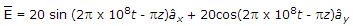Explanation: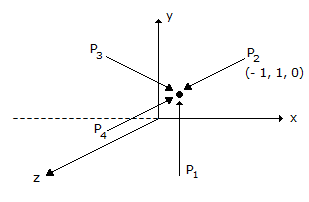E = 6.82ax + 6.82ay + 32.89az V/m

r = ax + ay + az

r1 = ax + ay

r2 = - ax + ay

r3 = - ax - ay

and r4 = ax - ay

r - r1 = az;

r - r2 = 2ax + az;

r - r3 = 2ax + 2ay + az

r - r4 = 2ay + az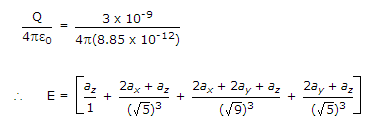= 6.8ax + 6.8ay + 32.9az .

27.

In the interconnection of ideal sources shown in the figure, it is known that the 60V source is absorbing power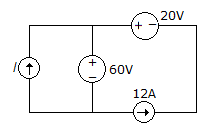Which of the following can be the value of the current source?

 A. 10 A B. 13 A C. 15 A D. 18 A

Explanation:

Since 12A current is coming from one source and it is also known that 60V source is absorbing power i.e. current is flowing inside 60V source.

12 = x + 1 => I = 12 - x.

28.

For the network shown below, if VD = 12 V and VGSQ = - 2 V value of RS will be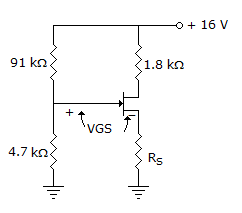A. 13.35 kΩ B. 3.35 kΩ C. 33.31 kΩ D. 3.95 kΩ

Explanation: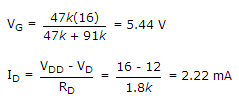VGS = VG - IDRS

- 2 = 5.44 - 2.22 X 10-3 X RS

RS = 3.35 KΩ.

29.

The causal system given below is __________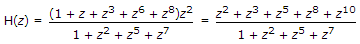A. minimum phase B. maximum phase C. mixed phase D. none of these

Explanation: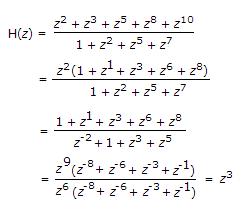It has only zeros at origin. Hence it is minimum phase system.

30.

Find RL for maximum power transfer.A. 3 Ω B. 1.125 Ω C. 4.1785 Ω D. None of these

Explanation:

Use Y - Δ transformation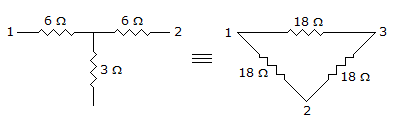RAB= (9 || 18) || (9 || 18) = 3 Ω.